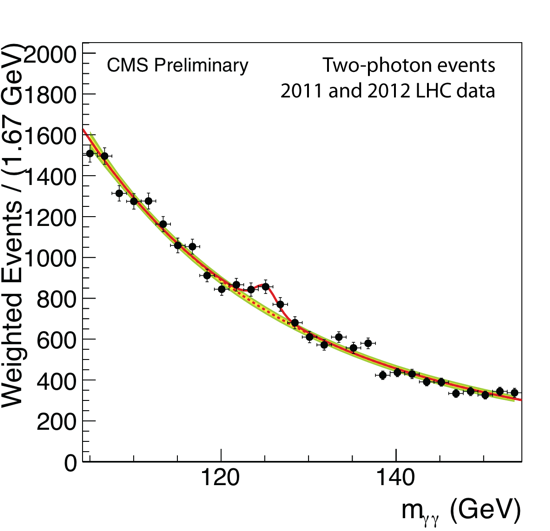## Wednesday, October 09, 2013

### I am Vindicated on my Prediction Eight Years Ago

Eight years ago, I predicted that there will be a resonance state at either 6*PI/alpha, or 4*PI/alpha:

http://quantoken.blogspot.com/2005/03/mass-of-top-quark-calculated.html

It comes out that 4*PI/alpha was correct. LHC claims the Higgs Boson discovery at 120 GeV. That energy level was predicted by me:The mass was at 4*PI/alpha. Alpha being the fine structure constant: 1/alpha = 137.03599911. So 4*PI/alpha = 1722.045.

The mass unit equals electron mass divided by alpha, or 137.03599911 times electron mass, or 137.03599911 * 0.510999 MeV = 70.0252585 MeV.

Thus the resonance state I predicted was 1722.045 * 70.0252585 MeV = 120.58666 GeV. That’s precisely where the “bump” occurs.

#### 2 Comments:Zephir said...

Hi, Quantoken, welcome back! I really do appreciate it!

Can you explain the underlying logics of your derivations/calculations?

9:14 AMZephir said...

BTW Randall Mills predicted mass of Higgs boson to 128.75 GeV

1:52 PM